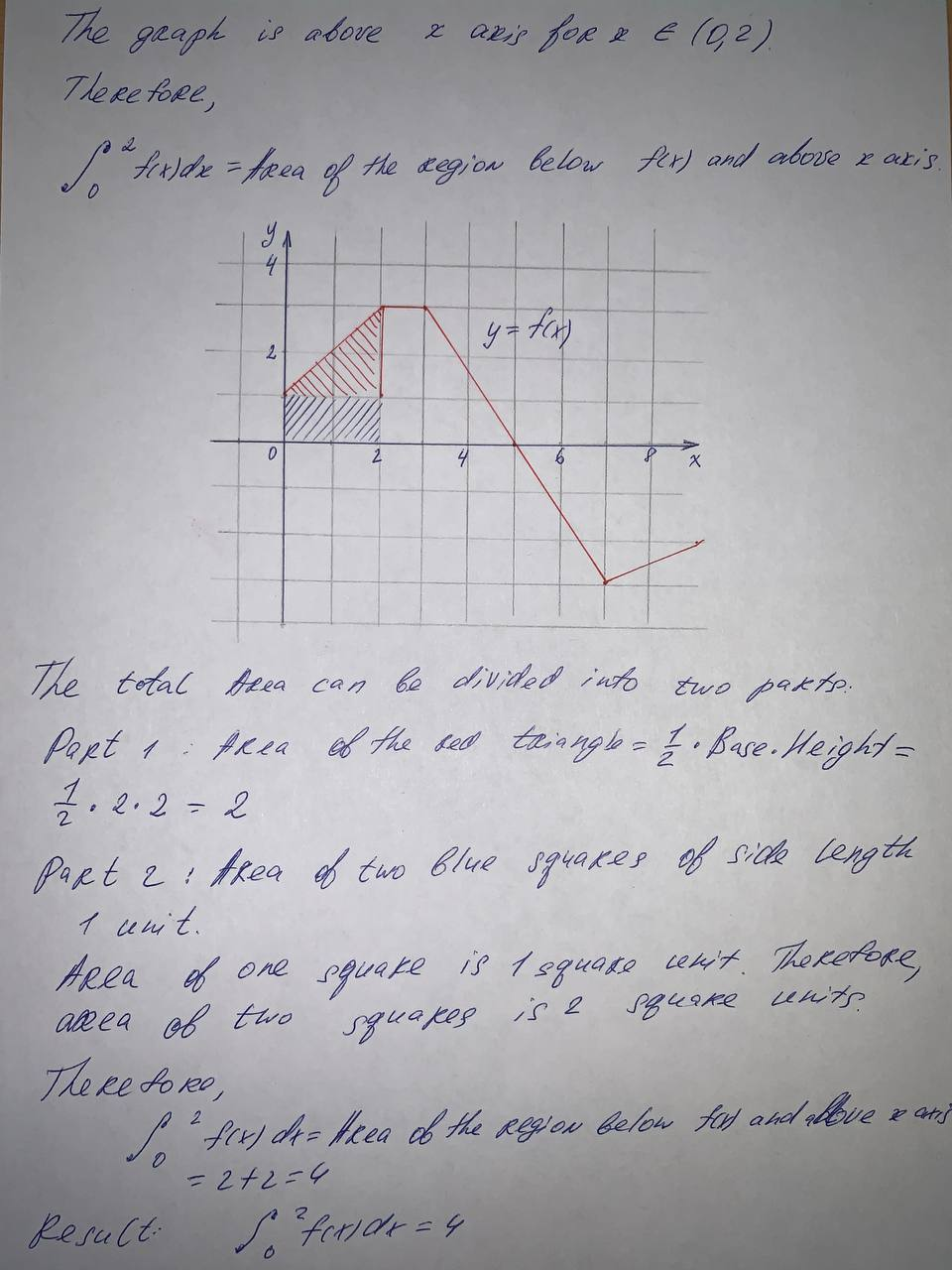#### Didn’t find what you are looking for?

Question# The graph of f is shown. Evaluate each integral by interpreting it in terms of areas. integral.\int_0^2 f(x)dx

Integrals
ANSWEREDThe graph of f is shown. Evaluate each integral by interpreting it in terms of areas. integral.$$\int_0^2 f(x)dx$$